# Insa Cremer – Strong semilattice decomposition of m-domain rings

University of Latvia
A semigroup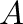is said to be a D-semigroup if there exists some subsetof its set of idempotents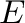such that, for every, there exists the smallestin the sense of the usual partial order of idempotents with the property that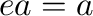. So on every D-semigroup, we can define a unary operation assigning to eachthe smallest idempotentwith.

An m-domain ring is a ringsuch that, for every element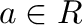, there exists a central idempotentsuch that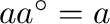,and, if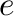is an idempotent such that, then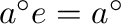. The multiplicative semigroup of an m-domain ring is a D-semigroup (with the unary operation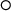).

Given an m-domain ring, we can decompose it into an inverse system of pairwise disjoint right-cancellative D-semigroups with identities over the set of idempotent elements of. In the talk, the following results on this decomposition will be presented.

First, the strong semilattice of D-semigroups obtained from this inverse system is the multiplicative D-semigroup of the ring.

Second, let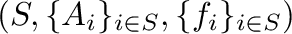be an inverse system of pairwise disjoint right-cancellative D-semigroups with identities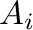over some lower semilattice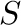. If the strong semilattice of D-semigroups obtained from the inverse systemis the multiplicative D-semigroup of some m-domain ring, then the inverse systemis the decomosition of the m-domain ring.